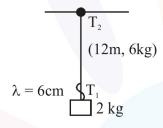# A uniform thin rope of length

Question:

A uniform thin rope of length $12 \mathrm{~m}$ and mass $6 \mathrm{~kg}$ hangs vertically from a rigid support and a block of mass $2 \mathrm{~kg}$ is attached to its free end. A transverse short wavetrain of wavelength $6 \mathrm{~cm}$ is produced at the lower end of the rope. What is the wavelength of the wavetrain (in $\mathrm{cm}$ ) when it reaches the top of the rope ?

1. 9

2.  12

3. 6

4. 3

Correct Option: , 2

Solution:$\mathrm{V} \propto \lambda \quad \mathrm{T}_{2}=8 \mathrm{~g}$

$\mathrm{T}_{1}=2 \mathrm{~g}$

$\frac{\mathrm{V}_{1}}{\mathrm{~V}_{2}}=\frac{\lambda_{1}}{\lambda_{2}}$

$\lambda_{2}=\frac{\mathrm{V}_{2}}{\mathrm{~V}_{1}} \lambda_{1}=\sqrt{\frac{\mathrm{T}_{2}}{\mathrm{~T}_{1}}} \times \lambda_{1}$

$=\sqrt{\frac{8 g}{2 g}} \lambda_{1}=2 \times 6=12 \mathrm{~cm}$